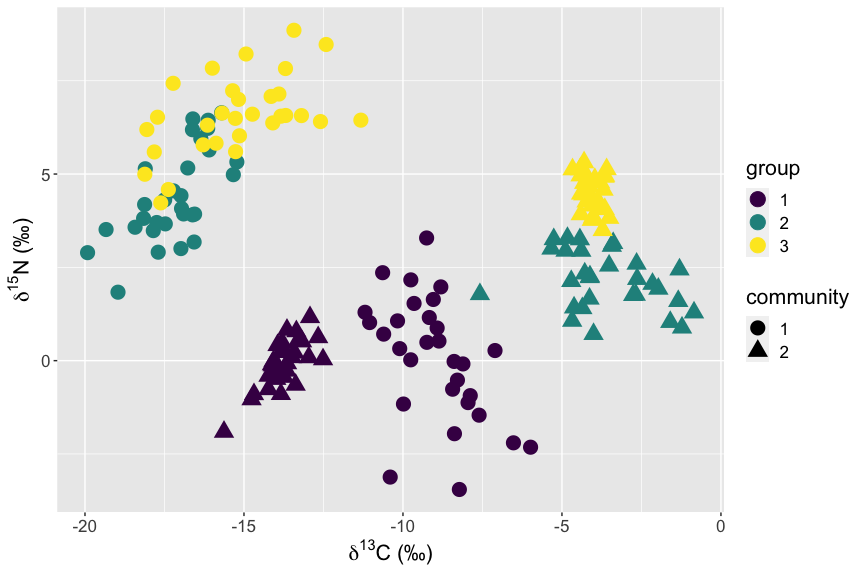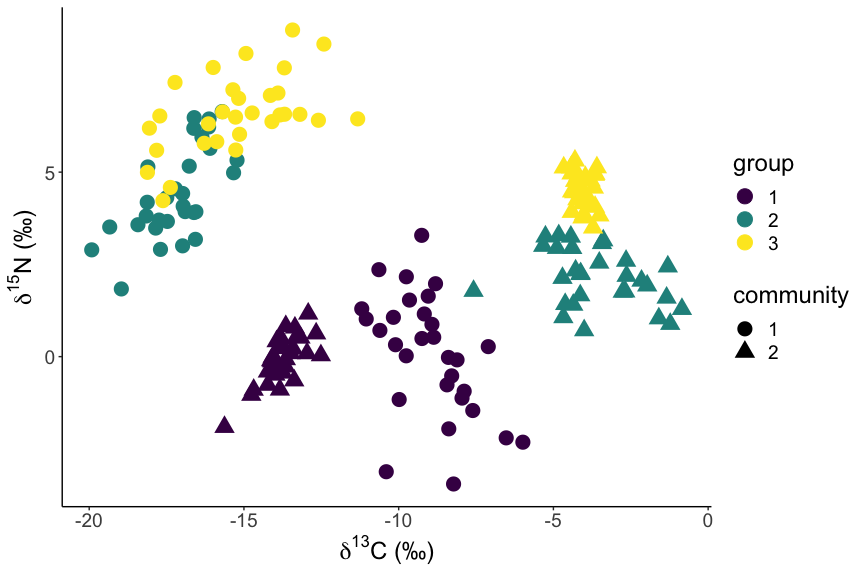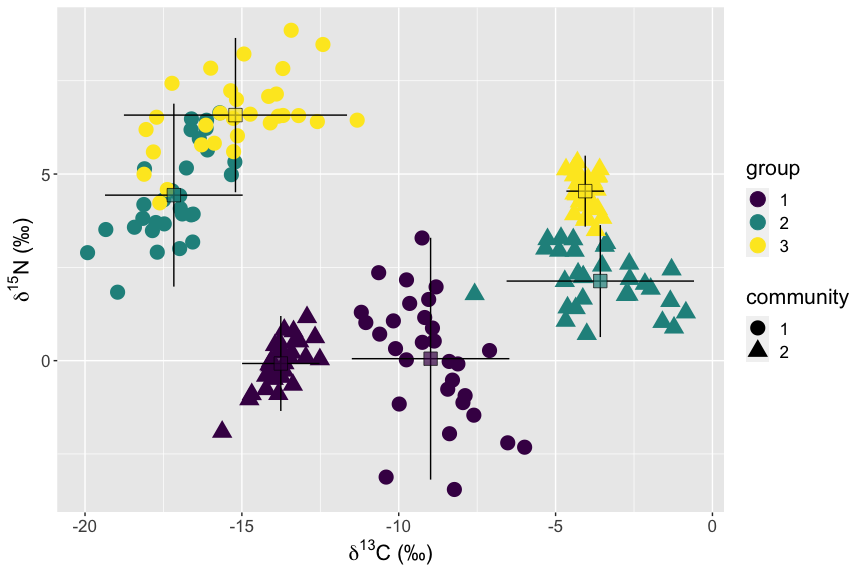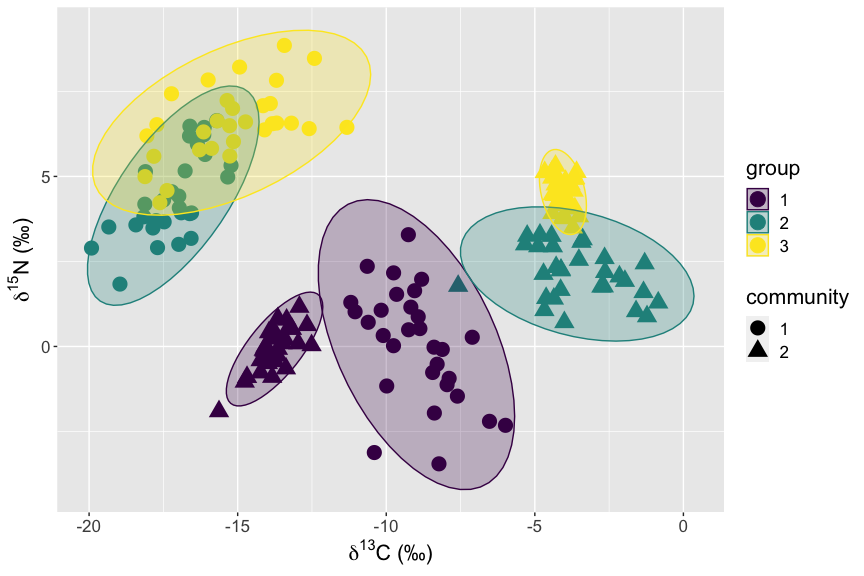# SIA plots using ggplot2

#### 2021-04-13

Import the data as before. Note that we get some warnings from both that “objects are masked from” various packages. This is because the packages we have just loaded have functions of the same name as those that have already been loaded (usually ones from the base R packages). This warning is telling us that, in the case of filter, when we simply call filter(), we will be using the last loaded one, i.e. from dplyr, rather than the one from stats. If you want to force R to use a particular function from a particular package you can write the long form, dplyr::filter() or stats::filter(). In many ways, it is good practice to always use this format, but in reality we are all lazy.

library(SIBER)
library(tidyverse)
## Registered S3 method overwritten by 'cli':
##   method     from
##   print.boxx spatstat.geom
## ── Attaching packages ─────────────────────────────────────── tidyverse 1.3.0 ──
## ✓ tibble  3.1.0     ✓ purrr   0.3.4
## ✓ tidyr   1.1.3     ✓ stringr 1.4.0
## ✓ readr   1.4.0     ✓ forcats 0.5.1
## ── Conflicts ────────────────────────────────────────── tidyverse_conflicts() ──
## x purrr::set_names() masks magrittr::set_names()
# import the data. Replace this line with a read.csv() or similar call
# to you own local file.
data("demo.siber.data")

# make a copy of our data for use here in this example, and
# set the columns group and community to be factor type using dplyr.
# Additionally rename the isotope data columns and drop the iso1 and iso2
# columns using the .keep option to keep only those that were not used to
# create the new variables, i.e. keep only ones on the left of the "=".
demo_data <- demo.siber.data %>% mutate(group = factor(group),
community = factor(community),
d13C = iso1,
d15N = iso2,
.keep = "unused") 

The graphics that are part of “base R” are not very pretty (or at least some people think so - I quite like them to be honest). Another one of Hadley Wickham’s very popular packages is ggplot2 which by default makes some very blog-friendly graphics. And of course you can change the theme (template) on them to get something more suitable for publication (and fashion seems to be changing here too).

In ggplot2, figures are created in layer, by first creating a basic layer of axes according to an “aesthetic” which is then used as a framework to add points and lines and other embellishments. If you push the layers into an object using the <- assignment as I have done here, then you will need to print() your figure to get it to render.

# when plotting colors and shapes, we need to tell ggplot that these are to
# be treated as categorical factor type data, and not numeric.
first.plot <- ggplot(data = demo_data,
aes(x = d13C,
y = d15N)) +
geom_point(aes(color = group, shape = community), size = 5) +
ylab(expression(paste(delta^{15}, "N (\u2030)"))) +
xlab(expression(paste(delta^{13}, "C (\u2030)"))) +
theme(text = element_text(size=16)) +
scale_color_viridis_d()

# And print our plot to screen
print(first.plot)If you want to use the more normal plotting format for journals without gridlines, and without the light-grey background, you add theme_classic() to your plot. This is a shortcut to some default settings of the myriad options available in theme() which we used above to increase the text size in our plot.

classic.first.plot <- first.plot + theme_classic() +
theme(text = element_text(size=18))

# and print to screen
print(classic.first.plot)# options to add to point the axis tick marks inwards
# theme(axis.ticks.length = unit(0.1, "cm"))

# Errorbar scatterplots

Adding the means and error bars in both directions to create the classic isotope scatterplot requires adding these as additional layers. We could re-write all the lines of code for the original plot above, or we can simply create a new ggplot object based on the original, and then add the layers we want, and print. We need some summary data for each of the groups to plot the intervals: we use dplyr::summarise as in the previous script today except this time we store the output into a new object which I call sbg. When we add the layers for the means and the errorbars, we need to tell the layers to use a new aesthetic mapping using the new x and y data: mapping = aes(x = mC, y = mN, ...).

# Summarise By Group (sbg)
sbg <- demo_data %>%
group_by(group, community) %>%
summarise(count = n(),
mC = mean(d13C),
sdC = sd(d13C),
mN = mean(d15N),
sdN = sd(d15N))
## summarise() has grouped output by 'group'. You can override using the .groups argument.
# make a copy of the first.plot object
# second.plot <- first.plot

# add the layers using the summary data in sbg
second.plot <- first.plot +
geom_errorbar(data = sbg,
mapping = aes(x = mC, y = mN,
ymin = mN - 1.96*sdN,
ymax = mN + 1.96*sdN),
width = 0) +
geom_errorbarh(data = sbg,
mapping = aes(x = mC, y = mN,
xmin = mC - 1.96*sdC,
xmax = mC + 1.96*sdC),
height = 0) +
geom_point(data = sbg, aes(x = mC,
y = mN,
fill = group),
color = "black", shape = 22, size = 5,
alpha = 0.7, show.legend = FALSE) +
scale_fill_viridis_d()
## Warning: Ignoring unknown aesthetics: x
print(second.plot)The ggplot2 function stat_ellipse allows us to easily add ellipses, of varying level which corresponds to the prediction interval. This function defaults to using the t-distribution so we will override this and specify the normal distribution as is consistent with the SIBER approach. Note that it is not possible to draw the small sample size corrected ellipses using this method: I need to create a new geom_ for SIBER and it is on my to-do list.

# use our ellipse function to generate the ellipses for plotting

# decide how big an ellipse you want to draw
p.ell <- 0.95

# create our plot based on first.plot above adding the stat_ellipse() geometry.
# We specify thee ellipse to be plotted using the polygon geom, with fill and
# edge colour defined by our column "group", using the normal distribution and
# with a quite high level of transparency on the fill so we can see the points
# underneath. In order to get different ellipses plotted by both columns "group"
# and "community" we have to use the interaction() function to ensure both are
# considered in the aes(group = XYZ) specification. Note also the need to
# specify the scale_fill_viridis_d() function as the mapping of colors for
# points and lines is separate to filled objects and we want them to match.
ellipse.plot <- first.plot +
stat_ellipse(aes(group = interaction(group, community),
fill = group,
color = group),
alpha = 0.25,
level = p.ell,
type = "norm",
geom = "polygon") +
scale_fill_viridis_d()

print(ellipse.plot)## Trouble shooting

If the permil symbol ‰ is not showing correctly (and instead is printing as $$\text{\u2030}$$ in your plot it is likely because your computer is not set up to use UTF-8 format character encoding. This is not a problem with your setup of R or Rstudio, but is deeper in your computer. It is fixed by changing the region or locale settings on your computer. You can access these in the system preferences area of your computer’s operating system. To check if your computer is set up to identify and interpret UTF-8 encoding, you can type sessionInfo() in the R console. You should see something like this: en_IE.UTF-8/en_IE.UTF-8/en_IE.UTF-8/C/en_IE.UTF-8/en_IE.UTF-8 under the heading “locale”. On my machine, this indicates that it is use english (en), for Ireland (IE) and the UTF-8 encoding format. If the UTF-8 format is missing from your sessionInfo() then you could try changing your operating system to a locale similar to your own region’s location and see if the UTF-8 format is supported there.